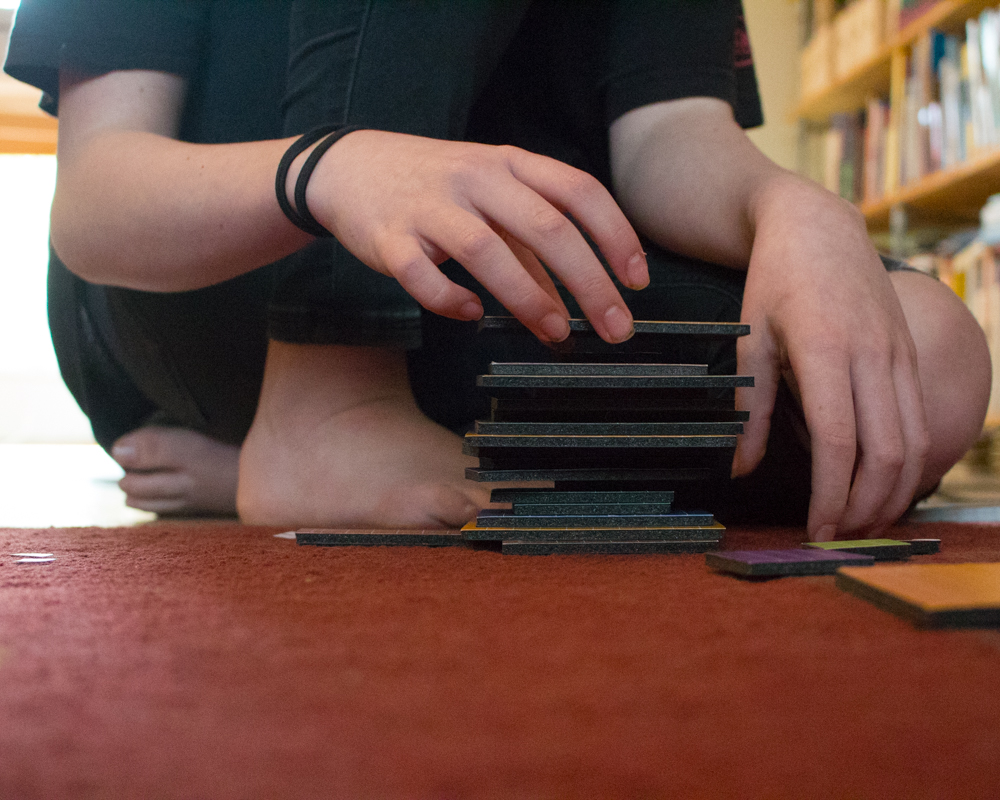## Saturday, April 25, 2020

### How to Square Binomials and Trinomials using Area Models

Let's say that you have two lengths: a and b. You would like to know what area would be covered by a square whose sides are each of these lengths combined.

The equation for that is (a+b)^2.

But how do you actually multiply that?

The algebraic way is to use the FOIL method: First, Outside, Inside, Last. This gives you (a+b)(a+b)=a^2 + ab + ab + b^2, which you simplify to (a+b)(a+b) = a^2 + 2ab + b^2.

That's fine algebraically, and you should totally memorize it, but here's what you should VISUALIZE when you do this, because here's what makes sense:

Visualize sitting on the rug in your family room. It's a Friday afternoon, soooooooo close to the end of your school week, and you'd very much rather be done with school and go walk your dog or listen to your music, but your mother wants to do one final project together before she sets you free. She hands you and your sister the decanomial square manipulatives and asks you to set them up:Stacking the area models is NOT part of setting them up, but is also nearly irresistable.

Fun fact: a decanomial square is the same concept as a binomial square or a trinomial square. Whereas a binomial square is (a+b)^2 and a trinomial square is (a+b+c)^2, a decanomial square is the representation of (a+b+c+d+e+f+g+h+i+j)^2. How would you like to factor THAT puppy without being able to visualize an area model to make sense of it?

Your mother asks you to choose two squares from the decanomial. You'll label one square as a^2, with sides measuring length a, and the other square as b^2, with sides measuring length b. The challenge is build an area model of a square whose sides are each length a+b; to complete this challenge you may use a^2, b^2, and two other rectangles of your choice.Visually, it's not hard to find the rectangles that match to complete the square, but it might take a little longer to notice that these rectangles each have two sides that match the length of one of the squares.

The solution to the puzzle, then, is (a+b)^2 = a^2 + ab + ab + b^2. Since you have two rectangles labeled ab, you can simplify your equation to (a+b)^2 = a^2 + 2ab + b^2.

This is exactly what you get when you the FOIL method, but doesn't it make a little more sense to see it?

Unfortunately, your cruel mother now tells you that you have to add a THIRD square to your puzzle. She. Is. So. MEAN!

You add a third square and label it c^2, with sides measuring length c. The puzzle is now a trinomial square, (a+b+c)^2, and your challenge is, once again, to complete the square. Your mother does not tell you how many pieces you have to use to complete this trinomial square, so you make yourself a shortcut:

Actually, this works algebraically!

The equation you've created is (a+b+c)^2 = a^2 + 2ab +b^2 + 2((a+b)c) + c^2. As long as you can complete the puzzle with area models that have sides that relate to lengths a, b, or c, you can translate the solution algebraically... but this isn't the simplest solution algebraically.

THIS is the puzzle solution that's also the simplest algebraically:

(a+b+c)^2 = a^2 + 2ab + b^2 + 2bc + c^2 + 2ac.

You can make infinitely bigger squares with an infinite number of terms. I mean, think of how many terms that decanomial square has, and yet it still follows the pattern you can see in the binomial and trinomial square.

And as soon as you memorize the binomial square and trinomial square equations that you created, you can go listen to music out on the back deck with your cat!